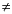Functions and CALL Routines

# FPUT Function

Moves data to the File Data Buffer (FDB) of an external file, starting at the FDB's current column position.
 Category: External Files

## Syntax

 FPUT(file-id,cval)

### Arguments

file-id

is a numeric variable that specifies the identifier that was assigned when the file was opened, generally by the FOPEN function.

cval

is a character constant, variable, or expression that specifies the file data.

FPUT returns 0 if the operation was successful,0 if it was not successful. The number of bytes moved to the FDB is determined by the length of the variable. The value of the column pointer is then increased to one position past the end of the new text.

Note:   You cannot write a new record in place of the current record if the new record has a length that is greater than the current record.This example assigns the fileref MYFILE to an external file and attempts to open the file in APPEND mode. If the file is opened successfully, indicated by a positive value in the variable FID, it moves data to the FDB using FPUT, appends a record using FWRITE, and then closes the file. Note that in a macro statement you do not enclose character strings in quotation marks.

```%macro ptest;
%let filrf=myfile;
%let rc=%sysfunc(filename(filrf,test.txt));
%let fid=%sysfunc(fopen(&filrf,a));
%if &fid > 0 %then
%do;
%let mystring=This is some data.;
%let rc=%sysfunc(fput(&fid,&mystring));
%let rc=%sysfunc(fwrite(&fid));
%let rc=%sysfunc(fclose(&fid));
%end;
%else
%put %sysfunc(sysmsg());
%let rc=%sysfunc(filename(filrf));
%put return code = &rc;
%mend;
%ptest;```

SAS writes the following output to the log:

`return code = 0`

 Functions:Previous Page | Next Page | Top of Page